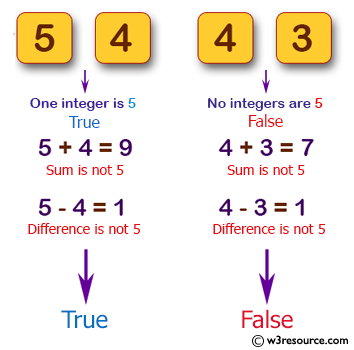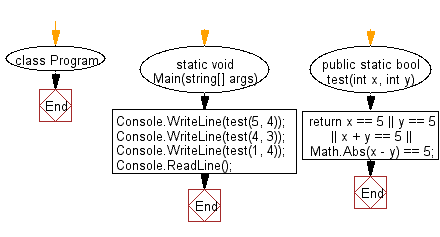﻿ C# - Check if either integer or their sum or difference is 5# C# Sharp Basic Algorithm Exercises: Accept two integers and return true if either one is 5 or their sum or difference is 5

## C# Sharp Basic Algorithm: Exercise-41 with Solution

Write a C# Sharp program that accept two integers and return true if either one is 5 or their sum or difference is 5.

Pictorial Presentation:Sample Solution:-

C# Sharp Code:

``````using System;
using System.Linq;
namespace exercises
{
class Program
{
static void Main(string[] args)
{
Console.WriteLine(test(5, 4));
Console.WriteLine(test(4, 3));
Console.WriteLine(test(1, 4));
}
public static bool test(int x, int y)
{
return x == 5 || y == 5 || x + y == 5 || Math.Abs(x - y) == 5;
}
}
}
```
```

Sample Output:

```True
False
True```

Flowchart:C# Sharp Code Editor:

Improve this sample solution and post your code through Disqus

What is the difficulty level of this exercise?

Test your Programming skills with w3resource's quiz.

﻿